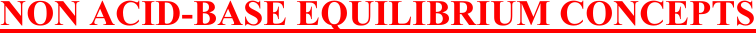Study Guides (380,000)
US (220,000)
Rutgers (3,000)
3:40 (100)
Final

01:160:161 Chapter Notes - Chapter 16: Joule, Endothermic Process, BromineExam

Department
Chemistry
Course Code
01:160:161
Professor
Asbed Vassilia
Study Guide
Final

This preview shows pages 1-3. to view the full 22 pages of the document.1
CHAPTER 16 (TRO)
NON ACID/BASE CHEMICAL EQUILIBRIUM
Problems to prepare students for hourly exam II.
Non acid-base equilibrium concepts
Non acid-base equilibrium calculations
Le Chatelier’s Principle
E. Tavss 2/17

Only pages 1-3 are available for preview. Some parts have been intentionally blurred.2
NON ACID-BASE EQUILIBRIUM CONCEPTS
Chem 162-2011 Hourly Exam II + Answers
Chapter 14 - Non Acid/Base Chemical Equilibrium
Non acid-base equilibrium concepts
13. 2NO(g) + Cl2(g) 2NOCl(g) Kp =1.40 x 105 at 298K
What is Kp at 298 K for the reaction: NOCl(g) NO(g) + ½ Cl2(g)
A. 7.14 x 10-6
B. 3.57 x 10-6
C. 1.34 x 10-3
D. 5.34 x 10-3
E. 2.67 x 10-3
Chem 162-2011 Hourly Exam II + Answers
Chapter 14 - Non Acid/Base Chemical Equilibrium
Non acid-base equilibrium concepts
10. Which of the following statements concerning the reaction quotient Q and/or the equilibrium
constant K is false?
A. Q must be equal to K at equilibrium.
B. The larger the value of K, the farther the forward reaction proceeds toward
completion.
C. As the temperature increases, the value of K increases.
D. The concentrations of pure solids and pure liquids are omitted from the
expression for Q, because they do not change during the course of a chemical
reaction.
E. By comparing the value of Q with K one can predict the direction of
change for a system not initially in equilibrium.

Only pages 1-3 are available for preview. Some parts have been intentionally blurred.

3
CHEM 162-2010 EXAM II
Chapter 14 - Non Acid/Base Chemical Equilibrium
Non acid-base equilibrium concepts
25. SO2Cl2(g)
SO2(g) + Cl2(g) K = 0.070
Flask X: 0.50 mol SO2Cl2, 0.10 mol SO2, 0.20 mol Cl2 in 0.50L
Flask Y: 0.50 mol SO2Cl2, 0.10 mol SO2, 0.20 mol Cl2 in 1.00L
Flask Z: 0.50 mol SO2Cl2, 0.10 mol SO2, 0.20 mol Cl2 in 2.00L
Which flask will result in a reaction forming more Cl2?
D. Flasks X and Y only
E. Flasks Y and Z only
CHEM 162-2010 EXAM II
Chapter 14 - Non Acid/Base Chemical Equilibrium
Non acid-base equilibrium concepts
16. Given: C(s) + CO2(g)
2CO Kp = 1.3 x 1014
COCl2(g)
CO(g) + Cl2(g) Kp = 167
Calculate Kp for: C(s) + CO2(g) + 2Cl2(g)
2COCl2
A. 2.2 x 1016
B. 7.8 x 1011
C. 1.3 x 1012
D. 2.6 x 1011
E. 4.7 x 109
CHEM 162-2010 EXAM II
Chapter 14 - Non Acid/Base Chemical Equilibrium
Non acid-base equilibrium concepts
6. Which of the following is true about a chemical reaction at equilibrium?
X. The rate of the forward reaction equals the rate of the reverse reaction.
Y. There is no observable change in any property of the system.
Z. The molecules have less kinetic energy than before equilibrium was reached.
A. X and Y only